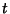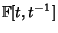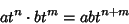## Laurent Polynomial

A Laurent polynomial with Coefficients in the Fieldis an algebraic object that is typically expressed in the formwhere theare elements of, and only finitely many of theare Nonzero. A Laurent polynomial is an algebraic object in the sense that it is treated as a Polynomial except that the indeterminant'' can also have Negative Powers.

Expressed more precisely, the collection of Laurent polynomials with Coefficients in a Fieldform a Ring, denoted, with Ring operations given by componentwise addition and multiplication according to the relationfor allandin the Integers. Formally, this is equivalent to saying thatis the Group Ring of the Integers and the Field. This corresponds to(the Polynomial ring in one variable for) being the Group Ring or Monoid ring for the Monoid of natural numbers and the Field.